# Writing a function rule given a table of ordered pairs: One-step rules Online Quiz

#### Excel Pivot Tables and Dashboard. Simple & Quick tutorial!

Most Popular

7 Lectures 58 mins

#### Learn HTML Tables

15 Lectures 59 mins

#### Excel Analytics - Data Analysis with Pivot-Tables and Charts

46 Lectures 2.5 hours

Following quiz provides Multiple Choice Questions (MCQs) related to Writing a function rule given a table of ordered pairs: One-step rules. You will have to read all the given answers and click over the correct answer. If you are not sure about the answer then you can check the answer using Show Answer button. You can use Next Quiz button to check new set of questions in the quiz.Q 1 - Given the following table of ordered pairs, write a one-step function rule.### Explanation

Step 1:

From the table 0 − 2 = −2; 1 − 2 = −1 and so on

Step 2:

Input – 2 = Output or x – 2 = y

Step 3:

Therefore the rule is ‘Subtract 2’ from the input to get the output.

Q 2 - Given the following table of ordered pairs, write a one-step function rule.### Explanation

Step 1:

From the table 0 × 3 = 0; 1 × 3 = 3 and so on

Step 2:

Input × 3 = Output or x × 3 = y

Step 3:

Therefore the rule is ‘Multiply by 3’ the input to get the output.

Q 3 - Given the following table of ordered pairs, write a one-step function rule.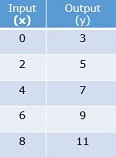### Explanation

Step 1:

From the table 0 + 3 = 3; 2 + 3 = 5 and so on

Step 2:

Input + 3 = Output or x + 3 = y

Step 3:

Therefore the rule is ‘Add 3’ to the input to get the output.

Q 4 - Given the following table of ordered pairs, write a one-step function rule.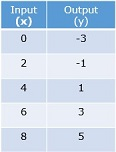### Explanation

Step 1:

From the table 0 − 3 = −3; 1 − 3 = −3 and so on

Step 2:

Input – 3 = Output or x – 3 = y

Step 3:

Therefore the rule is ‘Subtract 3’ from the input to get the output.

Q 5 - Given the following table of ordered pairs, write a one-step function rule.### Explanation

Step 1:

From the table 0 × 5 = 0; 1 × 5 = 5 and so on

Step 2:

Input × 5 = Output or x × 5 = y

Step 3:

Therefore the rule is ‘Multiply by 5’ the input to get the output.

Q 6 - Given the following table of ordered pairs, write a one-step function rule.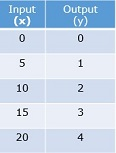### Explanation

Step 1:

From the table 0 ÷ 5 = 0; 5 ÷ 5 = 1 and so on

Step 2:

Input ÷ 5 = Output or x ÷ 5 = y

Step 3:

Therefore the rule is ‘Divide by 5’ the input to get the output.

Q 7 - Given the following table of ordered pairs, write a one-step function rule.### Explanation

Step 1:

From the table 0 − 1 = −1; 1 − 1 = 0 and so on

Step 2:

Input – 1 = Output or x – 1 = y

Step 3:

Therefore the rule is ‘Subtract 1’ from the input to get the output.

Q 8 - Given the following table of ordered pairs, write a one-step function rule.### Explanation

Step 1:

From the table 0 × 6 = 0; 1 × 6 = 6 and so on

Step 2:

Input × 6 = Output or x × 6 = y

Step 3:

Therefore the rule is ‘Multiply by 6’ the input to get the output.

Q 9 - Given the following table of ordered pairs, write a one-step function rule.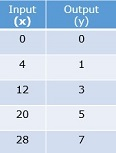### Explanation

Step 1:

From the table 0 ÷ 4 = 0; 4 ÷ 4 = 1 and so on

Step 2:

Input ÷ 4 = Output or x ÷ 4 = y

Step 3:

Therefore the rule is ‘Divide by 4’ the input to get the output.

Q 10 - Given the following table of ordered pairs, write a one-step function rule.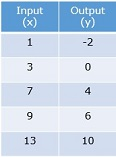### Explanation

Step 1:

From the table 0 − 3 = −3; 1 − 3 = −3 and so on

Step 2:

Input – 3 = Output or x – 3 = y

Step 3:

Therefore the rule is ‘Subtract 3’ from the input to get the output.

writing_function_rule_given_table_of_ordered_pairs_one_step_rules.htm